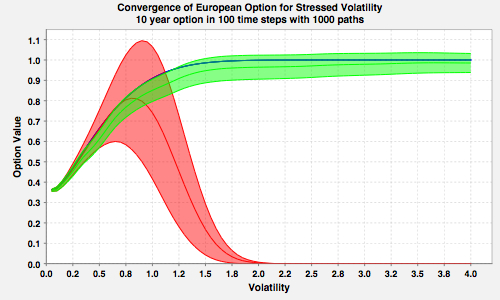# Monte-Carlo Simulations with Boundary Conditions

Fries, Christian P.; Kienitz, Jörg: Monte-Carlo Simulation with Boundary Conditions (with Applications to Stress Testing, CEV and Variance-Gamma Simulation). 2010.

### AbstractThe Monte-Carlo valuation (line) and its error estimate (corridor) for a European call option: Standard Monte-Carlo valuation (red), Monte-Carlo valuation with linear boundary condition (green) and analytic benchmark (blue).

In this paper we discuss how to incorporate analytic boundary conditions into a Monte-Carlo simulation framework and discuss their applications. The method introduced can dramatically improve the stability, robustness and accuracy of the valuation, calculation of sensitivities and stress testing, i.e., valuation under stressed model parameters.

We propose a Monte-Carlo simulation scheme which features boundary conditions for the underlying value process. The boundary conditions are analogous to the boundary conditions of a PDE. The Monte-Carlo simulation will then be modified to generate paths only within the boundaries and generate the corresponding Monte-Carlo weights. In addition, the valuation algorithm is adjusted to incorporate the analytic boundary conditions, using given or estimated boundary values for the value process.

The complete setup consists of four parts:

• The definition of a boundary and the corresponding in-bound and out-bound regime. This is done for each time step.
• The definition of a Monte-Carlo scheme for which all paths remain within the boundary.
• The definition of a boundary condition which defines the value process on the out-bound region and its valuation conditional to being in the in-bound region at the previous time-step.
• A modified pricing algorithm which allows to evaluate the product using the Monte-Carlo scheme within the boundary conditions, adding the boundary value process.
We present four different methods for calculating the product specific boundary value process: analytic, super-hedge, sub-hedge and numeric. Among the applications of the method we specifically lock at its behavior under stressed data.